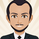จำนวนเข้าชม 3648
Adaptive Moving Average indicator script. This indicator was originally developed by Vitali Apirine (Stocks & Commodities V.36:5: Adaptive Moving Averages).
เอกสารเผยแพร่: Refactored
เอกสารเผยแพร่:
A list of Free indicators:
https://bit.ly/2S7EPuN

A list of Paid indicators:
https://bit.ly/33MA81f

Earn \$30:

Toss a coin to your witcher:
https://www.paypal.me/alexeverget

## ความคิดเห็นIs there anyway to convert this script, or atleast the ama(src, length, fastLength, slowLength) to Easy Language(for use with TradeStation)? I've been trying to no avail simply because I cannot find anything in their reference that mimics tradingview's (nz) function(Aka replace a nan value). Any help at all would be greatly appreciated.
ตอบกลับHi @everget this is a perfect script that I use all the time! is it possible to make it multi time frame? I tried but it returns an error message bellow:

"Cannot use a mutable variable as an argument of the security function."

the code I tried was:

res = input(title="Resolution", type=input.resolution, defval="360")

s1 = security(syminfo.tickerid, res, ama, gaps=true)

plot(s1, title="upper line",color=color.purple, transp=50)

Do you have a solution for that?
ตอบกลับ"

inputs:
Price( numericseries ),
EffRatioLength( numericsimple ),
FastAvgLength( numericsimple ), { this input assumed to be a constant >= 1 }
SlowAvgLength( numericsimple ) ; { this input assumed to be a constant >= 1 }

{ Eff = Efficiency }

variables:
NetChg( 0 ),
TotChg( 0 ),
EffRatio( 0 ),
ScaledSFSqr( 0 ),
SlowAvgSF( 2 / ( SlowAvgLength + 1 ) ),
FastAvgSF( 2 / ( FastAvgLength + 1 ) ),
SFDiff( FastAvgSF - SlowAvgSF ) ,
pds(0),
MLTP(0),
SSC(0);

pds = EffRatioLength + 1;
MLTP = AbsValue(Price - Lowest(low, pds)) - (highest(high, pds) - price) / (highest(high, pds) - lowest(low, pds));
SSC = MLTP * (FastAvgSF - SlowAvgSF) + SlowAvgSF;

"

This is what I have so far, when plugged into Tradestation along with an indicator to plot it the AMA comes out significantly different than the one on my chart from tradingview. Thanks in advance.
ตอบกลับHi,
Function: _AMA

// TASC APR 2018
// _AMA
// Function
// Vitali Apirine

inputs:
Periods( numericsimple ),
FastAvgLength( numericsimple ),
SlowAvgLength( numericsimple ) ;

variables:
PDS( Periods + 1 ),
FastSC( 2 / ( FastAvgLength + 1 ) ),
SlowSC( 2 / ( SlowAvgLength + 1 ) ),
SSC( 0 ),
CST( 0 ),
MLTP( 0 );

MLTP = AbsValue( ( Close - Lowest( Low, PDS ) )
- ( Highest( High, PDS ) - Close ))
/ ( Highest( High, PDS ) - Lowest( Low, PDS ) ) ;

SSC = MLTP * ( FastSC - SlowSC ) + SlowSC ;

CST = Square( SSC ) ;

if CurrentBar = 1 then
_AMA = Close + CST * ( Close - Close )
else
_AMA = _AMA + CST * ( Close - _AMA ) ;

--------------------------------------------------------------------

// TASC APR 2018
// Indicator
// Vitali Apirine

inputs:
Periods( 10 ),
FastAvgLength( 2 ),
SlowAvgLength( 30 ) ;

variables:
AMA( 0 ),
KAMA( 0 ) ;

AMA = _AMA( Periods, FastAvgLength,
SlowAvgLength ) ;
FastAvgLength, SlowAvgLength ) ;

Plot1( AMA, "AMA", Cyan ) ;
Plot2( KAMA, "KAMA", Magenta ) ;
ตอบกลับeverget
@everget, Thank you so much! I truly appreciate it and the speedy help.
ตอบกลับ